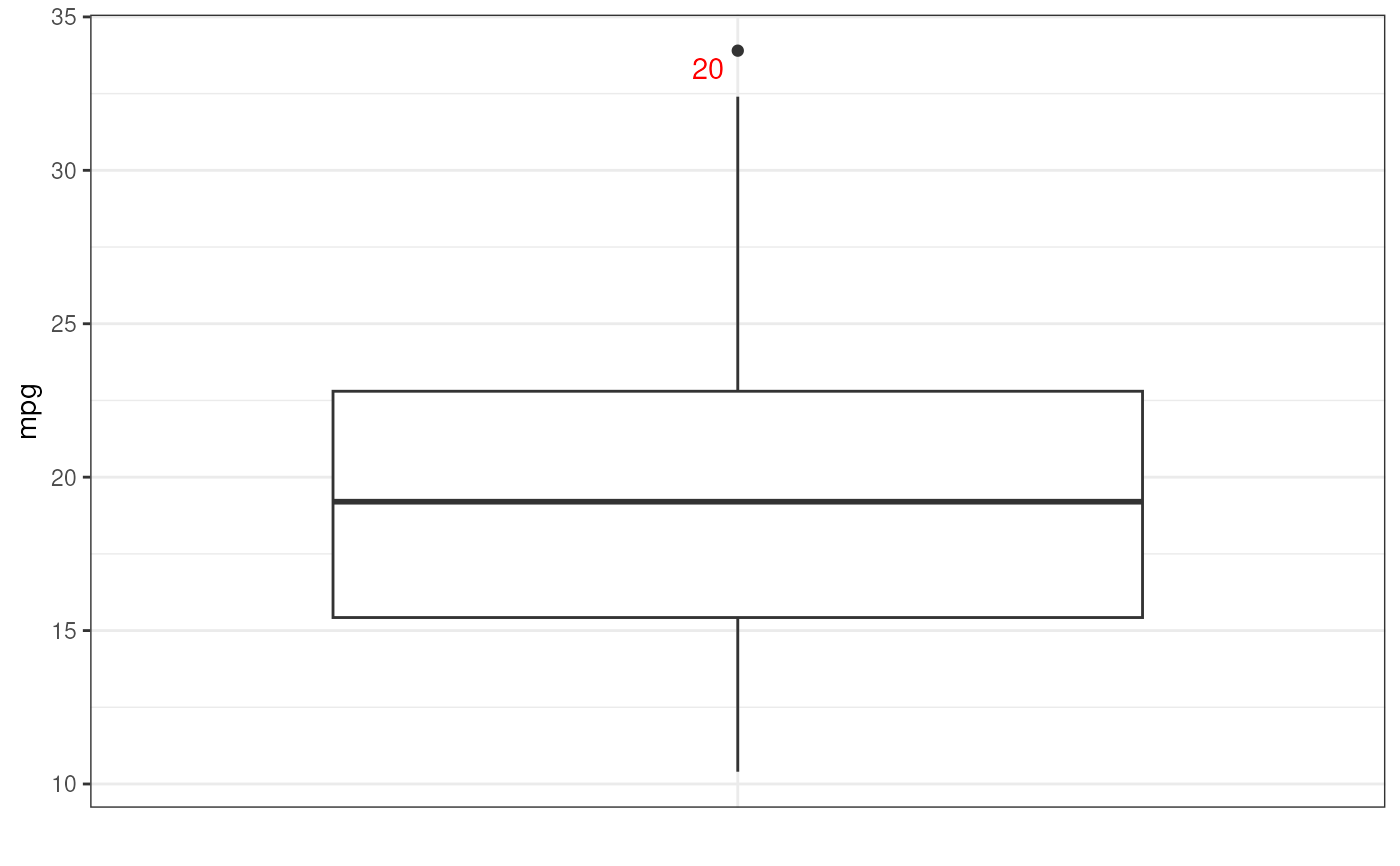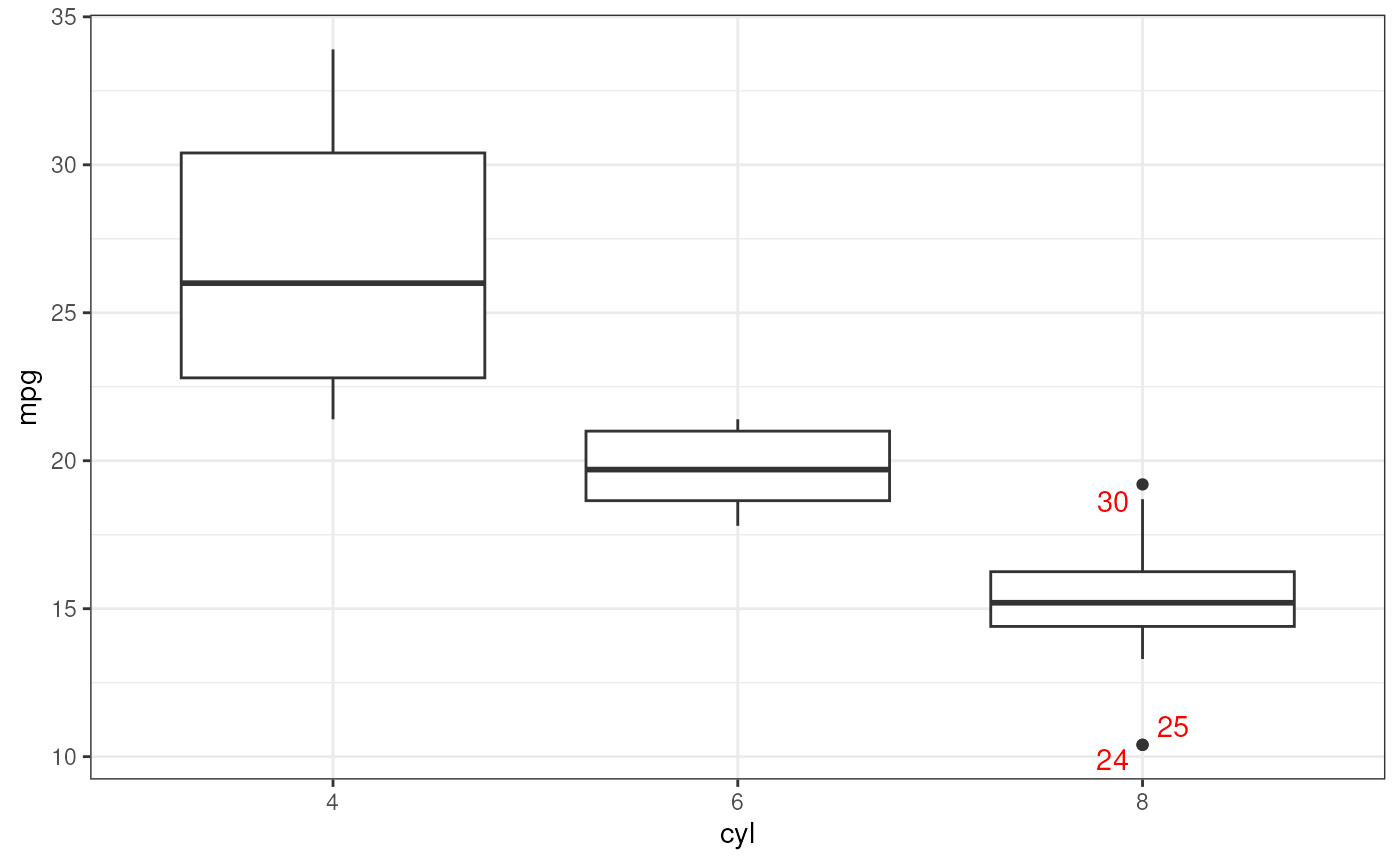This function provides a simple interface to create a ggplot box plot, organising different boxplots by levels of a factor is desired, and showing row numbers of outliers.

ggBoxplot(
dat,
y = NULL,
x = NULL,
labelOutliers = TRUE,
outlierColor = "red",
theme = ggplot2::theme_bw(),
...
)

## Arguments

dat

Either a vector of values (to display in the box plot) or a dataframe containing variables to display in the box plot.

y

If dat is a dataframe, this is the name of the variable to make the box plot of.

x

If dat is a dataframe, this is the name of the variable (normally a factor) to place on the X axis. Separate box plots will be generate for each level of this variable.

labelOutliers

Whether or not to label outliers.

outlierColor

If labeling outliers, this is the color to use.

theme

The theme to use for the box plot.

...

Any additional arguments will be passed to geom_boxplot.

## Value

A ggplot plot is returned.

## Details

This function is based on JasonAizkalns' answer to a question on Stack Exchange (Cross Validated; see https://stackoverflow.com/questions/33524669/labeling-outliers-of-boxplots-in-r).

geom_boxplot

## Author

Jason Aizkalns; implemented in this package (and tweaked a bit) by Gjalt-Jorn Peters.

Maintainer: Gjalt-Jorn Peters gjalt-jorn@userfriendlyscience.com

## Examples


### A box plot for miles per gallon in the mtcars dataset:
ggBoxplot(mtcars\$mpg);### And separate for each level of 'cyl' (number of cylinder):
ggBoxplot(mtcars, y='mpg', x='cyl');